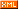# 沉于思考，默默学习！

:: :: :: :: :: :::: ::64 随笔 :: 0 文章 :: 714 评论 :: 0 引用

• ### 安全过滤后的getIP函数

  function getIP() {
$realip = ''; //设置默认值 if (isset($_SERVER['HTTP_X_FORWARDED_FOR'])) {
$realip =$_SERVER['HTTP_X_FORWARDED_FOR'];
} elseif (isset($_SERVER['HTTP_CLIENT_IP'])) {$realip = $_SERVER['HTTP_CLIENT_IP']; } else {$realip = $_SERVER['REMOTE_ADDR']; } preg_match('/^((?:\d{1,3}\.){3}\d{1,3})/',$realip,$match); return$match?$match:false; } 以上函数，增加了IP判断，只会读取以Ip格式数据开头，并且第一个满足IP格式值。如果没有返回false。 这样就可以读取到满足格式的IP，验证了数据的IP格式。 • ### 如果我读取互联网的IP，用户传入局域网的IP，我应该直接过滤掉 我们在一些网站上面，经常可以看到提示，非法的IP地址，其实一部分是IP地址格式错误，一部分可能是读取到IP地址，不满足互联网上面允许IP格式。 以下这个函数，是通过IANA站点规范，封装了个函数。 通过输入IP地址，能够准确知道，该IP是不是可以在互联网应用。 //互联网允许使用IP地址 function ipType2($ip) {
$iplist = explode(".",$ip);

if ($iplist >= 224 &&$iplist <= 239)
return '多播';
if ($iplist >= 240 &&$iplist <= 255)
return '保留';

if (preg_match('/^198\.51\.100/', $ip)) return 'TEST-NET-2，文档和示例'; if (preg_match('/^203\.0\.113/',$ip))
return 'TEST-NET-3，文档和示例';

if (preg_match('/^192\.(18|19)\./', $ip)) return '网络基准测试'; if (preg_match('/^192\.168/',$ip))
return '专用网络[内部网]';

if (preg_match('/^192\.88\.99/', $ip)) return 'ipv6to4中继'; if (preg_match('/^192\.0\.2\./',$ip))
return 'TEST-NET-1，文档和示例';
if (preg_match('/^192\.0\.0\./', $ip)) return '保留（IANA）'; if (preg_match('/^192\.0\.0\./',$ip))
return '保留（IANA）';

if ($iplist == 172 &&$iplist <= 31 && $iplist >= 16) return '专用网络[内部网]'; if ($iplist == 169 && $iplist == 254) return '链路本地'; if ($iplist == 127)
return '环回地址';
if ($iplist == 10) return '专用网络[内部网]'; if ($iplist == 0)
return '本网络（仅作为源地址时合法）';

return 'InterNet网地址';
}

posted on 2013-05-31 16:41  程默  阅读(4310)  评论(3编辑  收藏